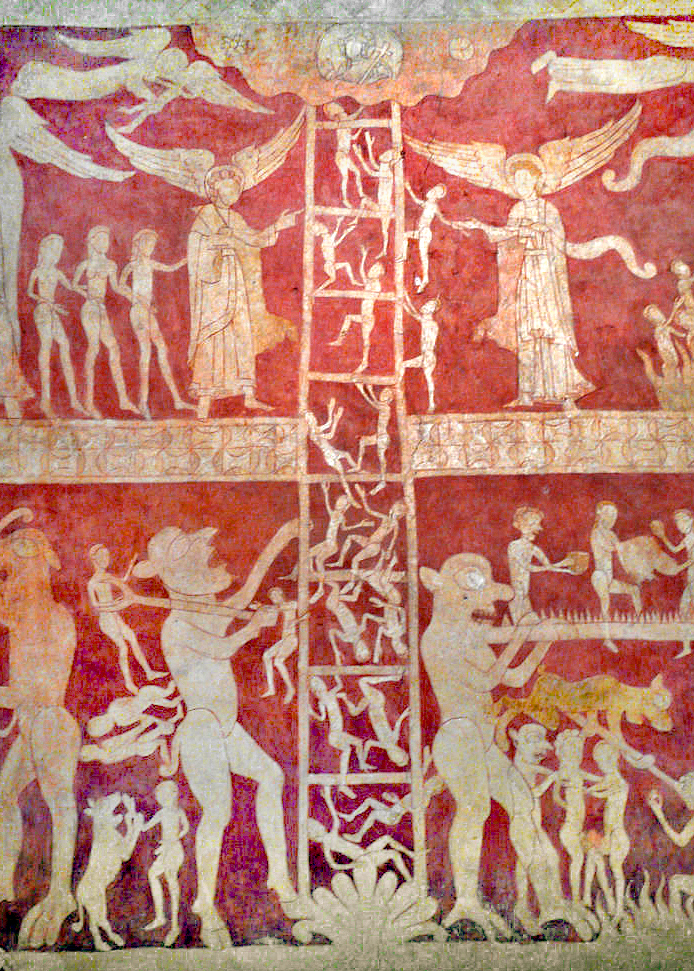# The axiom of well-ordered replacement is equivalent to full replacement over Zermelo + foundationIn recent work, Alfredo Roque Freire and I have realized that the axiom of well-ordered replacement is equivalent to the full replacement axiom, over the Zermelo set theory with foundation.

The well-ordered replacement axiom is the scheme asserting that if $I$ is well-ordered and every $i\in I$ has unique $y_i$ satisfying a property $\phi(i,y_i)$, then $\{y_i\mid i\in I\}$ is a set. In other words, the image of a well-ordered set under a first-order definable class function is a set.

Alfredo had introduced the theory Zermelo + foundation + well-ordered replacement, because he had noticed that it was this fragment of ZF that sufficed for an argument we were mounting in a joint project on bi-interpretation. At first, I had found the well-ordered replacement theory a bit awkward, because one can only apply the replacement axiom with well-orderable sets, and without the axiom of choice, it seemed that there were not enough of these to make ordinary set-theoretic arguments possible.

But now we know that in fact, the theory is equivalent to ZF.

Theorem. The axiom of well-ordered replacement is equivalent to full replacement over Zermelo set theory with foundation.

$$\text{ZF}\qquad = \qquad\text{Z} + \text{foundation} + \text{well-ordered replacement}$$

Proof. Assume Zermelo set theory with foundation and well-ordered replacement.

Well-ordered replacement is sufficient to prove that transfinite recursion along any well-order works as expected. One proves that every initial segment of the order admits a unique partial solution of the recursion up to that length, using well-ordered replacement to put them together at limits and overall.

Applying this, it follows that every set has a transitive closure, by iteratively defining $\cup^n x$ and taking the union. And once one has transitive closures, it follows that the foundation axiom can be taken either as the axiom of regularity or as the $\in$-induction scheme, since for any property $\phi$, if there is a set $x$ with $\neg\phi(x)$, then let $A$ be the set of elements $a$ in the transitive closure of $\{x\}$ with $\neg\phi(a)$; an $\in$-minimal element of $A$ is a set $a$ with $\neg\phi(a)$, but $\phi(b)$ for all $b\in a$.

Another application of transfinite recursion shows that the $V_\alpha$ hierarchy exists. Further, we claim that every set $x$ appears in the $V_\alpha$ hierarchy. This is not immediate and requires careful proof. We shall argue by $\in$-induction using foundation. Assume that every element $y\in x$ appears in some $V_\alpha$. Let $\alpha_y$ be least with $y\in V_{\alpha_y}$. The problem is that if $x$ is not well-orderable, we cannot seem to collect these various $\alpha_y$ into a set. Perhaps they are unbounded in the ordinals? No, they are not, by the following argument. Define an equivalence relation $y\sim y’$ iff $\alpha_y=\alpha_{y’}$. It follows that the quotient $x/\sim$ is well-orderable, and thus we can apply well-ordered replacement in order to know that $\{\alpha_y\mid y\in x\}$ exists as a set. The union of this set is an ordinal $\alpha$ with $x\subseteq V_\alpha$ and so $x\in V_{\alpha+1}$. So by $\in$-induction, every set appears in some $V_\alpha$.

The argument establishes the principle: for any set $x$ and any definable class function $F:x\to\text{Ord}$, the image $F\mathrel{\text{”}}x$ is a set. One proves this by defining an equivalence relation $y\sim y’\leftrightarrow F(y)=F(y’)$ and observing that $x/\sim$ is well-orderable.

We can now establish the collection axiom, using a similar idea. Suppose that $x$ is a set and every $y\in x$ has a witness $z$ with $\phi(y,z)$. Every such $z$ appears in some $V_\alpha$, and so we can map each $y\in x$ to the smallest $\alpha_y$ such that there is some $z\in V_{\alpha_y}$ with $\phi(y,z)$. By the observation of the previous paragraph, the set of $\alpha_y$ exists and so there is an ordinal $\alpha$ larger than all of them, and thus $V_\alpha$ serves as a collecting set for $x$ and $\phi$, verifying this instance of collection.

From collection and separation, we can deduce the replacement axiom $\Box$

I’ve realized that this allows me to improve an argument I had made some time ago, concerning Transfinite recursion as a fundamental principle. In that argument, I had proved that ZC + foundation + transfinite recursion is equivalent to ZFC, essentially by showing that the principle of transfinite recursion implies replacement for well-ordered sets. The new realization here is that we do not need the axiom of choice in that argument, since transfinite recursion implies well-ordered replacement, which gives us full replacement by the argument above.

Corollary. The principle of transfinite recursion is equivalent to the replacement axiom over Zermelo set theory with foundation.

$$\text{ZF}\qquad = \qquad\text{Z} + \text{foundation} + \text{transfinite recursion}$$

There is no need for the axiom of choice.